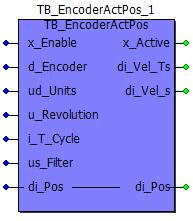## General Information

Generates the actual mechanical position in user units from the increment position of a connected encoder.

When x_Enable = TRUE, the module is activated. Successful activation is signaled with TRUE at the x_Active output. From this point, the angle changes of the connected encoder are detected, converted into user units, output and added to the position variable connected to di_Pos. The position should be set once by the user.

 Recommended task: EVENT Libraries used: ARITHMETIC_30bd00 or greater MOTION_CONTROL_xxbd00 or greater Additional data type definitions: ... Use of Static-Variables: ...

 none

## Input Parameter

Marking: Data type: Default: Data range: Description:
x_Enable BOOL ... ... TRUE: Enabling of the module.
d_Encoder DWORD ... ... Angle position of the encoder in increments. 232 increments correspond to one revolution of the encoder.
ud_Units UDINT ... ... Number of user units for the specified motor revolutions (u_Revolution).
u_Revolution UINT ... ... Number of encoder revolutions for the given user units (ud_Units).
ATTENTION:
The internal processing precision of the master axis depends on u_Revolution. The smaller the u_Revolution, the greater the resolution: u_Revolution          Resolution of the master axis
1                                                 30 bits
>= 2                                            29 bits
>= 4                                            28 bits
>= 8                                            27 bits
>= 16                                          26 bits
>= 32                                          25 bits
>= 2^x                                         32-(x+2) bits = 30-x bits
>= 4,096                                     18 bits
>= 8,192                                     17 bits
>= 16,384                                   16 bits
>= 32,768                                   15 bits
0 = 65,536                                  14 bits
i_T_Cycle INT ... ... Configured cycle time of setpoint event task in ms. 1, 2, 4 or 8 ms is possible.
us_Filter USINT ... ... Filter parameter for the angle change. Zero means filter inactive. The larger the value, the greater the filter effect.
di_Pos DINT ... ... Mechanical position of the encoder in user units.

## Output Parameter

Marking: Data type: Description:
x_Active BOOL TRUE: Module active; angle changes of the encoder are converted into user units and output.
di_Vel_Ts DINT Output of the current velocity in user units/Ts. Ts is the configured cycle time of the setpoint event task.
di_Vel_s DINT Output of the current velocity in user units/s.
di_Pos DINT Position of the encoder in user units